# Precalculus : Angles in the Coordinate Plane

## Example Questions

2 Next →

### Example Question #1 : Find The Measure Of A Coterminal Angle

Which of the following angles is coterminal to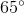?

Possible Answers: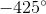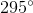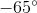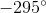Correct answer:Explanation:

Which of the following angles is coterminal to?

Coterminal angles are angles which start and end at the same point. In other words, they share both their starting and ending point. Note, this doesn't require them to be the same angle.

For instance,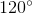is coterminal with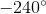, because they both start on the positive x-axis, and end at the same place in quadrant 2.

So, we want to find an angle that ends at the same place in quadrant 1 as. Of the answer choices, only 1 ends in quadrant 1, so that one must be our answer:2 Next →

### All Precalculus Resources